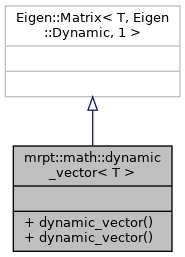MRPT  1.9.9
mrpt::math::dynamic_vector< T > Class Template Reference

## Detailed Description

### template<typename T> class mrpt::math::dynamic_vector< T >

Column vector, like Eigen::MatrixX*, but automatically initialized to zeros since construction.

Definition at line 44 of file eigen_frwds.h.

`#include <mrpt/math/eigen_frwds.h>`

Inheritance diagram for mrpt::math::dynamic_vector< T >:## Public Types

using Base = Eigen::Matrix< T, Eigen::Dynamic, 1 >

using mrpt_autotype = dynamic_vector< T >

using value_type = T

## Public Member Functions

dynamic_vector (size_t length=0)
Default constructor (vector of given size set to zero) More...

dynamic_vector (size_t length, float value)
Constructor to given size and all entries to some value. More...

## ◆ Base

template<typename T>
 using mrpt::math::dynamic_vector< T >::Base = Eigen::Matrix

Definition at line 70 of file types_math.h.

## ◆ mrpt_autotype

template<typename T>
 using mrpt::math::dynamic_vector< T >::mrpt_autotype = dynamic_vector

Definition at line 71 of file types_math.h.

## ◆ value_type

template<typename T>
 using mrpt::math::dynamic_vector< T >::value_type = T

Definition at line 72 of file types_math.h.

## ◆ dynamic_vector() [1/2]

template<typename T>
 mrpt::math::dynamic_vector< T >::dynamic_vector ( size_t length = `0` )
inline

Default constructor (vector of given size set to zero)

Definition at line 76 of file types_math.h.

## ◆ dynamic_vector() [2/2]

template<typename T>
 mrpt::math::dynamic_vector< T >::dynamic_vector ( size_t length, float value )
inline

Constructor to given size and all entries to some value.

Definition at line 78 of file types_math.h.

 Page generated by Doxygen 1.8.14 for MRPT 1.9.9 Git: 7d5e6d718 Fri Aug 24 01:51:28 2018 +0200 at lun nov 2 08:35:50 CET 2020